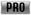## FANDOM

5,204 Pages

This is the {{Extrapolate pro upgrade price a8}} template. It can extrapolate an unknown price forupgrades of Asphalt 8 vehicles.

The template is heavily used by {{Pro upgrades table v4.2 a8}} to autocomplete upgrade tables, but can also be used by editors to calculate a single desired price.

It can handle numbers with thousands separators (e.g. 1,250), but the output is always unformatted (1250).

## Price progression

Level Factor
10 levels 5 levels car 5 levels bike
1 1 1 1
2 2 2 2
3 2.5 3 4
4 3.5 6.5 8
5 4 12.5 16
6 8
7 8.5
8 15.5
9 16
10 32

The template relies on the underlying price progressions that have been introduced with the 2019 Holiday Update (v4.7). The progressions for 5- and 10-level upgrades are shown in the table to the right.

Note: If Gameloft changes these progressions in the future, all calculactions done by this template become wrong!

Examples:

The upgrade price of a 10-level vehicle for

• level 2 will be 2 times the price of level 1
• level 10 will be 32 times the price of level 1.

The upgrade price of a 5-level car for

• level 3 will be 3 times the price of level 1
• level 5 will be 12.5 times the price of level 1.

Vice versa, the upgrade price of a 10-level vehicle for

• Level 1 will be the price of level 10 divided by 32 multiplied by 1.
• Level 7 will be the price of level 9 divided by 16 multiplied by 8.5.

The template searches the array `l1`...`l10` of given upgrade prices starting with `l1` and takes the first existing price it can find to calculate the requested price.

## Usage

```{{Extrapolate pro upgrade price a8
| levels =
| level =
| bike =
| l1 =
| l2 =
| l3 =
| l4 =
| l5 =
| l6 =
| l7 =
| l8 =
| l9 =
| l10 =
| <default>
}}
```

## Parameters

`levels`

• Required. The number of upgrade levels the vehicle has.
• Number: 5 or 10. Other values are ignored.

`level`

• Required. The level of the price to be calculated.
• Number between 1 and 10, <= `levels`. Numbers > `levels` are ignored.

`bike`

• Required if the vehicle is a bike. Caused the different price progression for bikes to be used.
• `1` or `yes` if the vehicle is a bike.

`l1`, `l2`, ... `l10`

• Optional. Existing prices for the respective leves.
• Number, with or without thousands separators.

`<default>`

• Optinal. The default value to be returned if no price can be calculated (mainly when there are no values for `l1`, `l2`, ... `l10` at all). If omitted, nothing is returned when calculation fails.
• Any text.

## Examples

### Main

• 10-level vehicle
• desired: level 1
• known: level 10 (320, so level 1 will be 320 / 32)

`{{Extrapolate pro upgrade price a8 | levels = 10| level = 1| l10 = 320}}` → 10

• 5-level vehicle
• desired: level 1
• known: level 5 (1,250, so level 1 will be 1,250 / 12.5)

`{{Extrapolate pro upgrade price a8 | levels = 5| level = 1| l5 = 1,250}}` → 100

• 5-level vehicle
• desired: level 4
• known: level 5 (1,250, so level 4 will be 1,250 / 12.5 * 6.5)

`{{Extrapolate pro upgrade price a8 | levels = 5| level = 4| l5 = 1,250}}` → 650

### Other

• Only the first level found (`l4`) is used to calculate, so the value of `l5` has no effect.
 ```{{Extrapolate pro upgrade price a8 | levels = 5 | level = 1 | l4 = 650 | l5 = 1,250,000,000 }} ``` → 100
 ```{{Extrapolate pro upgrade price a8 | levels = 5 | level = 1 | l4 = 650,000,000 | l5 = 1,250 }} ``` → 100000000
• no values to extrapolate from

`{{Extrapolate pro upgrade price a8 | levels = 10| level = 1}}`

• no values, but default output specified

`{{Extrapolate pro upgrade price a8 | levels = 10| level = 1| Meh.}}` → Meh.

• desired level higher than the total number of levels

`{{Extrapolate pro upgrade price a8 | levels = 5| level = 8| l5 = 1250}}`

Community content is available under CC-BY-SA unless otherwise noted.